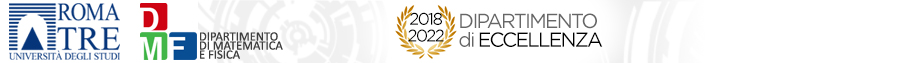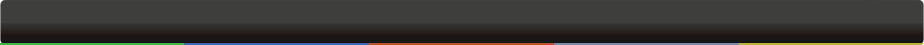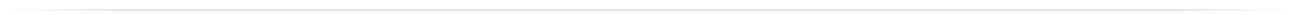# Seminari del Dipartimento

 AnalisiA rigidity theorem for an overdetermined problemMarcello Lucia03-07-2018 - 15:00Largo San Leonardo Murialdo,1 - Pal.C - Aula 211 On a bounded simply connected domain of $mathbb R^N$,  consider the Laplacian eigenvalue problem with overdetermined boundary conditions, where both constant Dirichlet and zero Neumann boundary are prescribed. This overdetermined problem admits a solution for instance in a disc, as can be seen by considering Neumann radial eigenfunctions. A conjecture due to Schiffer states that the ball is in fact the only possible domain where such problem can be solved. In dimension three, this rigidity theorem has recently been obtained by Ramm, but remains open in other dimensions. In this talk we will discuss this type of overdetermined condition in dimension two  for a general semilinear problem. We show that a rigidity theorem holds if in addition some higher normal derivatives are constant along the boundary.  This is a joint work with Bernd Kawohl (University of Cologne).org: ESPOSITO PierpaoloCopyright© 2014 Dipartimento di Matematica e Fisica